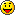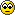## Geometry

Share your ZGE-development tips and techniques here!

Moderator: Moderators

Kjell
Posts: 1724
Joined: Sat Feb 23, 2008 11:15 pm

### GeometryA collection of useful ( and not so useful ) procedurally generated meshes. All generated with a Grid2DOnly MeshBox using U and V coordinates ranging from -Pi to Pi ( unless notified ).

First up, a diamond cluster.

Code: Select all

``````this.V.X = (2+sin(U)*sin(V*4))*sin(V)*-1;
this.V.Y = (2+sin(U)*sin(V*4))*cos(V);
this.V.Z = cos(U)*sin(V*4);``````
AttachmentsCluster.jpg (42.13 KiB) Viewed 14972 times
Last edited by Kjell on Tue Sep 30, 2008 3:00 pm, edited 1 time in total.

Kjell
Posts: 1724
Joined: Sat Feb 23, 2008 11:15 pm
Next, a teardrop.

Code: Select all

``````this.V.X = 0.5*(1-cos(U))*sin(U)*cos(V);
this.V.Y = 0.5*(1-cos(U))*sin(U)*sin(V);
this.V.Z = cos(U);``````
AttachmentsTeardrop.jpg (32.03 KiB) Viewed 14959 times

Kjell
Posts: 1724
Joined: Sat Feb 23, 2008 11:15 pm
Followed by, a pillow.

Code: Select all

``````this.V.X = cos(U);
this.V.Y = cos(V);
this.V.Z = (sin(U)*sin(V))/Pi*2;``````
AttachmentsPillow.jpg (34.56 KiB) Viewed 14958 times

Kjell
Posts: 1724
Joined: Sat Feb 23, 2008 11:15 pm
Let's try a spring.

Code: Select all

``````this.V.X = (Pi+Radius*cos(V))*cos(U*Length);
AttachmentsSpring.jpg (48.05 KiB) Viewed 14957 times

jph_wacheski
Posts: 1005
Joined: Sat Feb 16, 2008 8:10 pm
Contact:
Cool! thanks for posting these,. more fun stuff to play around with!
The more I work with these generative type geometries the better understanding I am developing of the math that defines them,. it is just great to have some starting places for gaining that.

I am wondering if we had a torus prim. if we could get rid of that seam on that very usefull torus bit you post elsewere? You know so the primitve, to begin with, was a solid torus geometry,. and we could use the equations to warp it from there? peace.
iterationGAMES.com

Kjell
Posts: 1724
Joined: Sat Feb 23, 2008 11:15 pm
By request, a torus with calculated normals ( unusual subdivisions to illustrate normals ).

Code: Select all

``````this.V.X = cos(U)*cos(V)*Size+cos(U)*Radius;
this.V.Z = sin(V)*Size;

this.N.X = cos(U)*cos(V)*Size;
this.N.Y = sin(U)*cos(V)*Size;
this.N.Z = this.V.Z;``````
AttachmentsTorus.jpg (55.17 KiB) Viewed 14922 times

kattle87
Posts: 402
Joined: Wed Sep 26, 2007 9:06 am
Location: Italy
I'm posting here also the Sphere and the cylinder with subdivided upper and lower faces. A cylinder is to come if someone ask for itNote: for the cylinder, you can change the variable "VarQ" that indicates how many "circles" you are going to have on upper and lower surface.

For Ville: try using "int" instead of "float" for VarP and tell me if it's working for you. I get different results. Is this because of truncate on integer divisions? If yes, it's fineAttachments
Sphere_Cylinder.zgeproj
In the fall of 1972 President Nixon announced that the rate of increase of inflation was decreasing. This was the first time a sitting president used the third derivative to advance his case for reelection.
-=Hugo Rossi=-

jph_wacheski
Posts: 1005
Joined: Sat Feb 16, 2008 8:10 pm
Contact:
Excellent! These are both great and usefull!! I apretiate the normal correctness, for perfect smoothing.

Keep 'em coming,. can you design a routine for a Icosphere?!?!

Hay,. I was just wundering about using renderMesh components to buils animated object,. would they be faster to animate? can closed shapes be made using these techneques??

I'm still looking for a way to do nice poly-trails,. you know 3d ribbons,. that fade in width,. and alpha preferably,. . I can get the points and build a list in arrays but havent figured how to draw them,.
iterationGAMES.com

Kjell
Posts: 1724
Joined: Sat Feb 23, 2008 11:15 pmUsing this one myself all the time ( for collision representation ).

Code: Select all

``````ZZDC<?xml version="1.0" encoding="iso-8859-1" ?>
<Mesh Name="CapsuleMesh">
<Producers>
<MeshBox Scale="0.5 0.5 1" XCount="15" Grid2DOnly="255"/>
<MeshExpression AutoNormals="0">
<Expression>
<![CDATA[float RX, SX, CX;

RX = this.V.X*PI*2;

SX = sin(RX); CX = cos(RX);

this.V.X = SX/2;
this.V.Z = CX/2;

this.N.X = this.V.X;
this.N.Y = 0;
this.N.Z = this.V.Z;]]>
</Expression>
</MeshExpression>
<MeshBox Scale="0.5 0.5 1" XCount="15" YCount="3" Grid2DOnly="255"/>
<MeshExpression AutoNormals="0">
<Expression>
<![CDATA[float RX, SX, CX,
RY, SY, CY;

RX = this.V.X*PI*2;
RY = this.V.Y*PI/2-PI/4;

SX = sin(RX); CX = cos(RX);
SY = sin(RY); CY = cos(RY);

this.V.X = SX*CY/2;
this.V.Y = SY/2;
this.V.Z = CX*CY/2;

this.N.X = this.V.X;
this.N.Y = this.V.Y;
this.N.Z = this.V.Z;

this.V.Y -= 0.5;]]>
</Expression>
</MeshExpression>
<MeshCombine/>
<MeshBox Scale="0.5 0.5 1" XCount="15" YCount="3" Grid2DOnly="255"/>
<MeshExpression AutoNormals="0">
<Expression>
<![CDATA[float RX, SX, CX,
RY, SY, CY;

RX = this.V.X*PI*2;
RY = this.V.Y*PI/2+PI/4;

SX = sin(RX); CX = cos(RX);
SY = sin(RY); CY = cos(RY);

this.V.X = SX*CY/2;
this.V.Y = SY/2;
this.V.Z = CX*CY/2;

this.N.X = this.V.X;
this.N.Y = this.V.Y;
this.N.Z = this.V.Z;

this.V.Y += 0.5;]]>
</Expression>
</MeshExpression>
<MeshCombine/>
</Producers>
</Mesh>``````
I'm aware you can generate a capsule out of a single plane as well, but this mesh is easier to modifyAttachmentsCapsule.jpg (17.88 KiB) Viewed 14337 times

jph_wacheski
Posts: 1005
Joined: Sat Feb 16, 2008 8:10 pm
Contact:
Very cool,. this reminded me that the mesh component now has the nifty Graph feature! Curious, how have you have implemented Capsule Collision? Can it be added to the standard ZGE collision tests or is it dependent on a bunch of your own code?
iterationGAMES.com

Kjell
Posts: 1724
Joined: Sat Feb 23, 2008 11:15 pmUsing my own collision libraries .. couldn't wait for that Raycast ( and the rest ) foreverK

diki
Posts: 140
Joined: Thu Sep 11, 2008 7:53 pm
Location: GMT+1
Contact:
Kjell wrote:my own collision libraries
ach, the envy!jph_wacheski
Posts: 1005
Joined: Sat Feb 16, 2008 8:10 pm
Contact:
I still suck with mesh math,. anyone able to generate this more correctly??

Code: Select all

``````ZZDC<?xml version="1.0" encoding="iso-8859-1" ?>
<Mesh Name="g_Z">
<Producers>
<MeshBox Grid2DOnly="255"/>
<MeshExpression>
<Expression>
<![CDATA[//V : current vertex
//N : current normal (turn off AutoNormals when modifying normals)
//C : current color (turn on VertexColors)
//TexCoord : current texture coordinate (turn on HasTexCoords)

v.y*=0.035;
v.x*=0.5;
v.x+=0.019;
v.y+=.385;]]>
</Expression>
</MeshExpression>
<MeshBox Grid2DOnly="255"/>
<MeshExpression>
<Expression>
<![CDATA[//V : current vertex
//N : current normal (turn off AutoNormals when modifying normals)
//C : current color (turn on VertexColors)
//TexCoord : current texture coordinate (turn on HasTexCoords)

v.x*=0.07;

if (v.y<-.5) v.y+=.65;
if (v.y<-.25) v.x-=.45;
if (v.y>.5) v.y-=.65;
if (v.y>.25) v.x+=.45;]]>
</Expression>
</MeshExpression>
<MeshCombine/>
<MeshBox Grid2DOnly="255"/>
<MeshExpression>
<Expression>
<![CDATA[//V : current vertex
//N : current normal (turn off AutoNormals when modifying normals)
//C : current color (turn on VertexColors)
//TexCoord : current texture coordinate (turn on HasTexCoords)

v.y*=0.035;
v.x*=0.5;
v.x-=0.019;
v.y-=.385;]]>
</Expression>
</MeshExpression>
<MeshCombine/>
<MeshTransform Scale="1 1.3 1"/>
</Producers>
</Mesh>
``````
iterationGAMES.com

Kjell
Posts: 1724
Joined: Sat Feb 23, 2008 11:15 pm
Hi jph,
jph_wacheski wrote:I still suck with mesh math, anyone able to generate this more correctly??
The letter "Z" isn't really a geometric primitive .. however, you could do something like this.

Code: Select all

``````ZZDC<?xml version="1.0" encoding="iso-8859-1" ?>
<Mesh Name="Mesh">
<Producers>
<MeshBox Scale="2 0.5 1" XCount="3" Grid2DOnly="255"/>
<MeshExpression AutoNormals="0">
<Expression>
<![CDATA[//

float Y = this.V.Y;

switch(this.V.X+2+(0.5-Y)*5)
{
case 0:
case 9:
this.V.Y *=  4;
break;

case 1:
case 8:
this.V.X *= -2;
this.V.Y *=  4;
break;

case 2:
case 7:
this.V.X  =  Y*4;
this.V.Y *=  3;
break;

case 3:
case 6:
this.V.X *= -1.2;
this.V.Y *= -3;
break;

case 4:
case 5:
this.V.Y *= -3;
break;
}]]>
</Expression>
</MeshExpression>
</Producers>
</Mesh>``````
K

jph_wacheski
Posts: 1005
Joined: Sat Feb 16, 2008 8:10 pm